## Filters

Sort by :
Clear All
Q

14.  In the adjoining figure, name the following pairs of angles.

(i) Obtuse vertically opposite angles
(iii) Equal supplementary angles
(iv) Unequal supplementary angles
(v) Adjacent angles that do not form a linear pair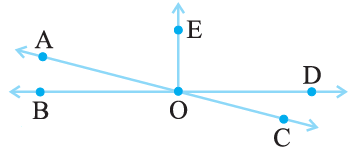(i)   are the vertically obtuse angles.  (ii)   are the complementary angles. (iii)   are the equal supplementary angles. (iv)   are the unequal pair of supplementary angle. (v)  ,   and   are adjacent angles but are not supplementary angles.

13.  Fill in the blanks:

(i) If two angles are complementary, then the sum of their measures is _______.

(ii) If two angles are supplementary, then the sum of their measures is ______.

(iii) Two angles forming a linear pair are _______________.

(iv) If two adjacent angles are supplementary, they form a ___________.

(v) If two lines intersect at a point, then the vertically opposite angles are always _____________.

(vi) If two lines intersect at a point, and if one pair of vertically opposite angles are acute angles, then the other pair of vertically opposite angles are  __________.

(i)      (ii)     (iii)   Supplementary angles (iv)   Straight line (v)     equal (vi)   obtuse angles (as they form a line).

12.(ii)  Find the values of the angles x, y, and z in each of the following:From the figure we can observe that :  (i)                       (Vertically opposite angle) (ii)             (Linear pair/straight line) (iii)          (Vertically opposite angle).

12.(i) Find the values of the angles x, y, and z in each of the following: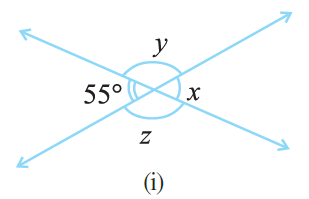From the figure : (i)             (Vertically opposite angle) (ii)      (Linear pair) (iii)                     (Vertically opposite angle)

10.(ii)  Indicate which pairs of angles are:

(ii) Linear pairs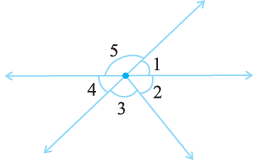The sum of angles in linear pair is . Thus the linear pairs are :    (a)     (b)

10.(i)   Indicate which pairs of angles are:

(i) Vertically opposite angles.The vertically opposite pairs are :               (a)    and                 (b)    and

11. In the following figure, is adjacent to ? Give reasons.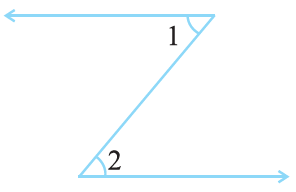No,   and    are not adjacent angles as their vertex is not same/common. For being adjacent angles the pair must have a common vertex and have a common arm.

(iii) Do  and  form a linear pair?
(iv) Are  and  supplementary?
(v) Is  vertically opposite to  ?
(vi) What is the vertically opposite angle of  ?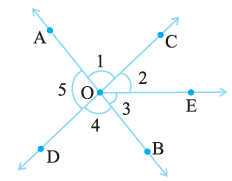(i) Yes,  adjacent to  as these have the same vertex and have one common arm. (ii)  No,   is not adjacent to . This is because  contains . (iii) Yes the given angles form a linear pair as they are pair of supplementary angles. (iv) Since BOA is a straight line thus the given angles are supplementary. (v) Yes,  and  are vertically opposite angles as they are the angles formed by two intersecting...

8. An angle is greater than . Is its complementary angle greater than or equal to or less than ?

We know that the sum of two complementary angles is  . Thus if one of the angles is greater than  then the other angle needs to be less than .

7.  Can two angles be supplementary if both of them are:

(i) acute ?   (ii) obtuse ?   (iii) right ?

We know that the sum of supplementary angles is . (i) The maximum value of the sum of two acute angles is less than . Thus two acute angles can never be supplementary. (ii) The minimum value of the sum of two obtuse angles is more than . Thus two obtuse angles can never be supplementary. (iii) Sum of two right angles is . Hence two right angles are supplementary.

6.  In the given figure, and are supplementary angles. If is decreased, what changes should take place in so that both the angles still remain supplementary.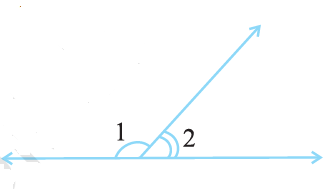Since it is given that  and  are supplementary angles, i.e. the sum of both angles is . Thus if  is decreased then to maintain the sum  needs to be increased.

5.  Find the angle which is equal to its supplement.

Let the required angle be . Then according to the question :                                                      or                                                            or                                                               Hence the angle is .

4. Find the angle which is equal to its complement.

Let the required angle be . Then according to question, we have :                                                       or                                                             or

3. Identify which of the following pairs of angles are complementary and which are supplementary.

We know that the sum of supplementary angles is   and the sum of complementary angle is . (i) Sum of the angles is :    . Hence these are supplementary angles. (ii) Sum of the angles is :    . Hence these are complementary angles. (iii) Sum of the angles is :    . Hence these are supplementary angles. (iv) Sum of the angles is :    . Hence these are supplementary angles. (v) Sum of the angles...

2.(iii)  Find the supplement of each of the following angles:We know that the sum of angles of supplementary pair is . Thus the supplement of the given angle is :

2.(ii) Find the supplement of each of the following angles:We know that the sum of angles of supplementary pair is . Thus the supplement of the given angle is :

2.(i)   Find the supplement of each of the following angles: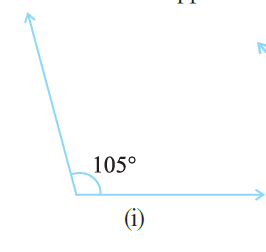We know that sum of supplement angles is   The supplement of the given angle is :

1.(iii)  Find the complement of each of the following angles: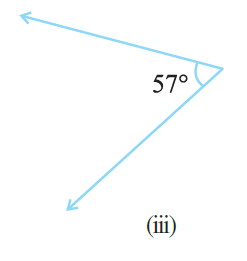The sum of complement angles is . Thus the complement of the angle is given by :

1.(ii)  Find the complement of each of the following angles: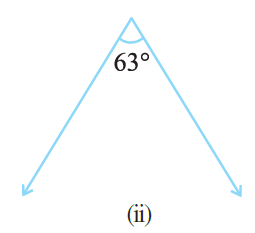The sum of the complementary angle is . Thus the complementary angle to the given angle is :

1.(i)  Find the complement of each of the following angles: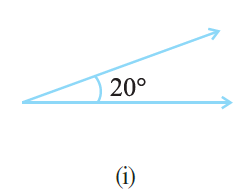The sum of complementary angle is . Thus the complementary angle to the given angle is :
Exams
Articles
Questions